# Maxwell distribution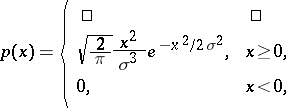(*)
depending on a parameter. The distribution function of the Maxwell distribution has the form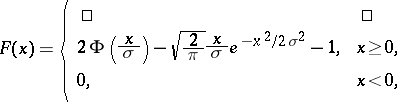where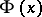is the standard normal distribution function. The Maxwell distribution has positive coefficient of skewness; it is unimodal, the unique mode occurring at. The Maxwell distribution has finite moments of all orders; the mathematical expectation and variance are equal to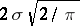and, respectively.
If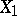,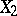andare independent random variables having the normal distribution with parametersand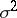, then the random variable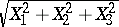has a Maxwell distribution with density (*). In other words, a Maxwell distribution can be obtained as the distribution of the length of a random vector whose Cartesian coordinates in three-dimensional space are independent and normally distributed with parametersand. The Maxwell distribution with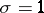coincides with the distribution of the square root of a variable having the-distribution with three degrees of freedom (see also Rayleigh distribution). The Maxwell distribution is widely known as the velocity distribution of particles in statistical mechanics and physics. The distribution was first defined by J.C. Maxwell (1859) as the solution of the problem on the distribution of velocities of molecules in an ideal gas.